﻿ 质量车蒸汽弹射系统仿真研究
«上一篇文章快速检索 高级检索

 哈尔滨工程大学学报2018, Vol. 39Issue (12): 1979-1986  DOI: 10.11990/jheu.2017080060

### 引用本文ZHU Qidan, LU Peng, YANG Zhibo. Simulation research on deadload steam catapult systems[J]. Journal of Harbin Engineering University, 2018, 39(12), 1979-1986. DOI: 10.11990/jheu.201708006.### 文章历史

Simulation research on deadload steam catapult systems
ZHU Qidan, LU Peng , YANG Zhibo
College of Automation, Harbin Engineering University, Harbin 150001, China
Abstract: To study the dynamics and thermodynamic process feature of the steam catapult system of aircraft carrier, simulation models were developed for the whole catapult process, which comprised tensioning, and catapult force built up with holdback and power stroke. This simulation was realized by setting up a thermodynamics model for steam catapult, mathematical model for traction release device, and kinetics model for deadload steam catapult system. According to three main parameters of accumulator pressure, cylinder pressure, and deadload acceleration, theoretical analysis and simulation were conducted, and then the correctness of the proposed models was validated by comparison with experimental data. The other main parameters for steam catapult system were studied using the model setup. From the simulation results, three important conclusions were obtained:Among the three kinds of resistances of the steam catapult system, the friction force of the deadload has the greatest influence, and air drag has the least influence. The curve of catapult force against the catapult stroke can be regarded as a piecewise function, whereby the slopes of the initial and latter segments are positive and negative, respectively. The peak value of the axial load of launch bar is greater than that of the catapult force. The study result can give a theory reference to design and test of steam catapult launch system.
Keywords: deadload    steam catapult system    catapult launch    whole process modeling of catapult    simulation of catapult whole process    whole process analysis of catapult

1 质量车蒸汽弹射系统及弹射过程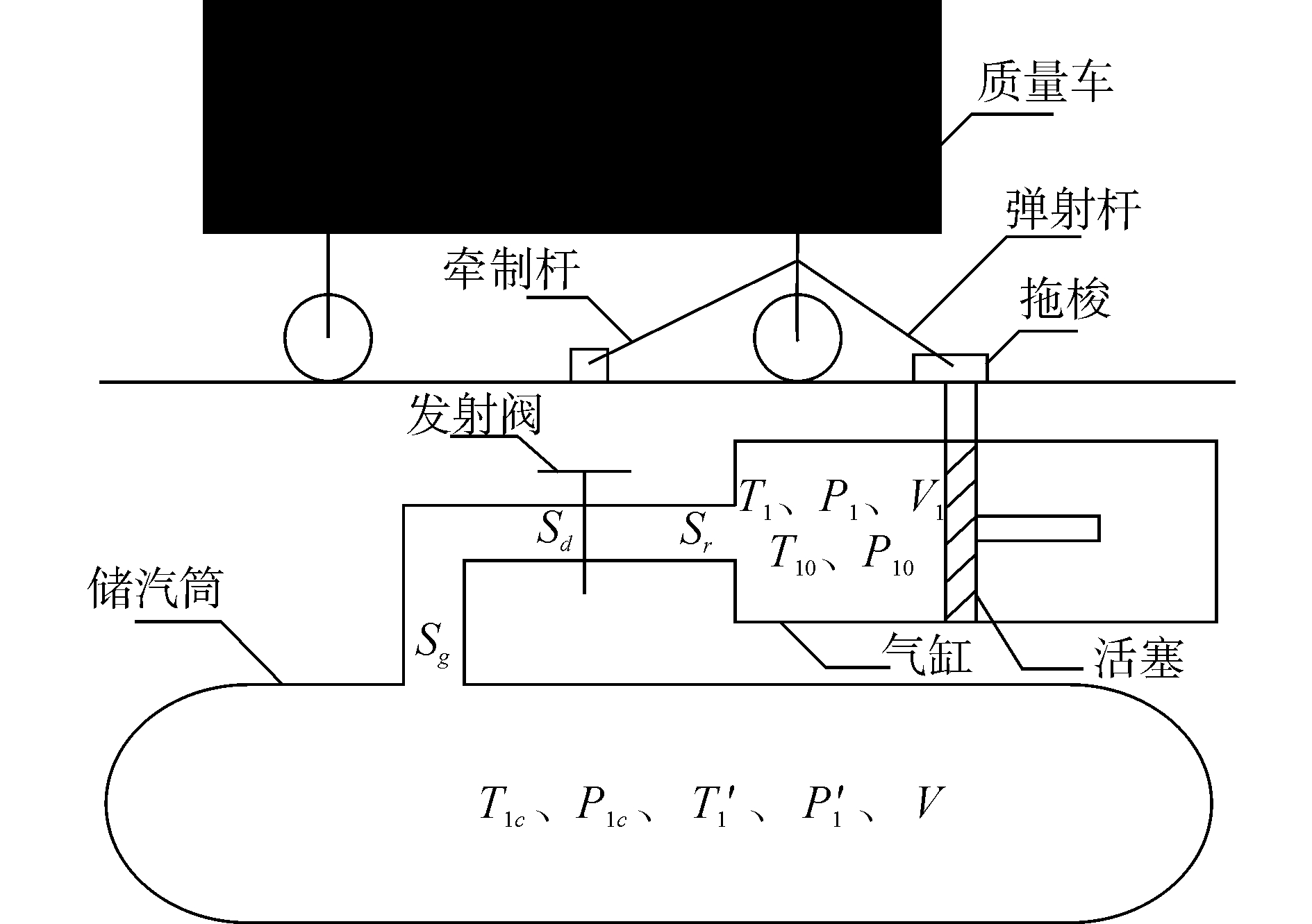Download: 图 1 质量车蒸汽弹射系统示意图 Fig. 1 The schematic of deadload steam catapult system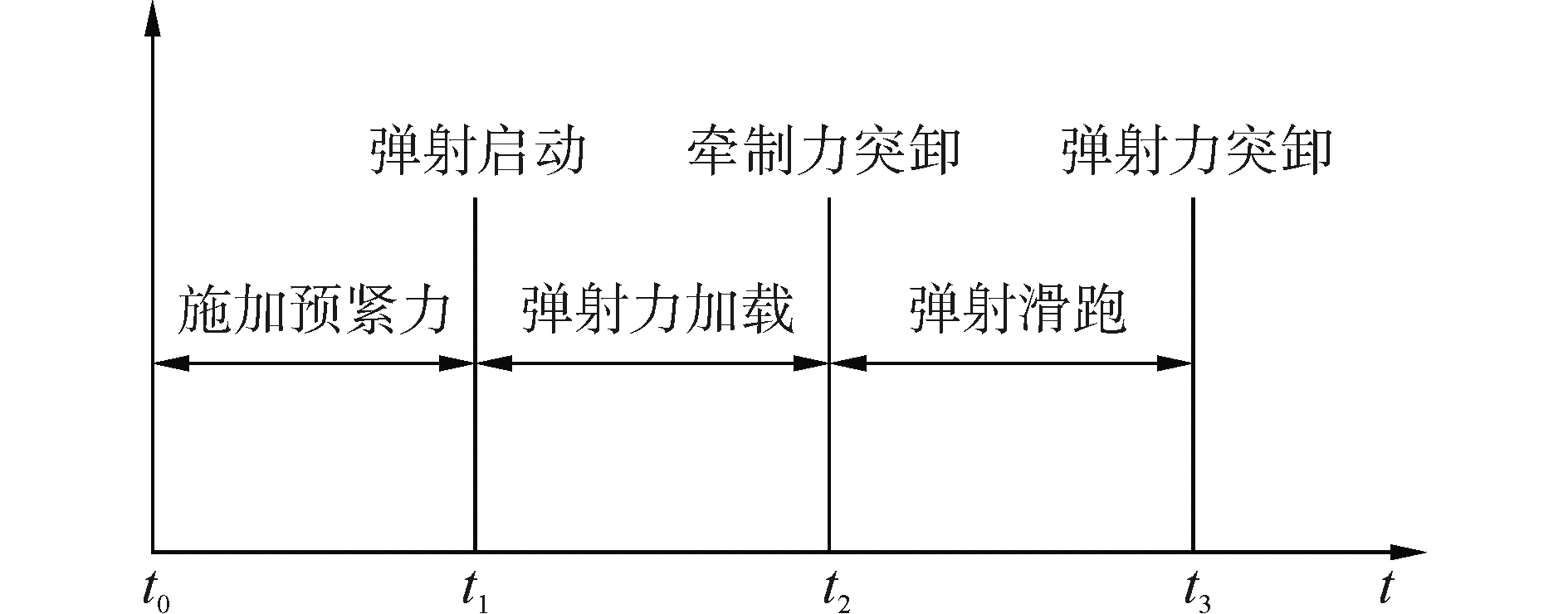Download: 图 2 蒸汽弹射仿真阶段划分 Fig. 2 Phases of steam catapult
2 质量车蒸汽弹射系统数学模型建立 2.1 蒸汽弹射器热力学模型

2.1.1 储汽筒模型

1) 在系统工作过程中，将储汽筒放汽的过程假定为绝热放汽过程，由于储汽筒放汽过程持续时间约为3 s，假设在此过程中储汽筒与外界不存在热交换；

2) 储汽筒为刚性容器，忽略压强和温度导致的体积变化。

3) 储汽筒放汽过程为准静态过程，可以近似认为在每一时刻，储汽筒内热力学变化量都无限接近于一平衡态，满足状态方程。

4) 忽略储汽筒放汽过程中，高温高压蒸汽与储汽筒壁之间的摩擦损失。

5) 认为在储汽筒和气缸之间的管道中，高温高压蒸汽做绝热稳定流动。

 ${{T'}_1} = {T_{1c}}{\left( {\frac{{{P_{1c}}}}{{{{P'}_1}}}} \right)^{\frac{{1 - \kappa }}{\kappa }}}$ (1)

 ${Q_{m1}} = \left\{ \begin{array}{l} {A_q}\frac{{{{P'}_1}}}{{\sqrt {{{T'}_1}} }}\sqrt {\frac{{2\kappa }}{{\kappa - 1}}\frac{1}{{{R_g}}}\left( {{{\left( {\frac{{{P_1}}}{{{{P'}_1}}}} \right)}^{\frac{2}{\kappa }}} - {{\left( {\frac{{{P_1}}}{{{{P'}_1}}}} \right)}^{\frac{{\kappa + 1}}{\kappa }}}} \right)} \\ \;\;\;\;\;\;\;\;\;\;\;\;\;\;\;\;\;\;\;\;\;\;\;\;\;\;\;\;\;\;\;\;\;\;\;\;{P_1} > {P_k}\\ {A_q}\frac{{{{P'}_1}}}{{\sqrt {{{T'}_1}} }}\sqrt {{{\left( {\frac{2}{{\kappa + 1}}} \right)}^{\frac{{\kappa + 1}}{{\kappa - 1}}}}\frac{\kappa }{{{R_g}}}} \;\;\;\;{P_1} \le {P_k} \end{array} \right.$ (2)

 ${P_k} = {{P'}_1} \times {\left( {\frac{2}{{\kappa + 1}}} \right)^{\frac{\kappa }{{\kappa - 1}}}}$ (3)

 $S = \frac{1}{{\sqrt {\frac{1}{{S_g^2}} + \frac{1}{{S_d^2}} + \frac{1}{{S_r^2}}} }}$ (4)

 ${{i'}_1}{\rm{d}}{M_s} = - {\rm{d}}{U_1}$ (5)

 ${\rm{d}}{U_1} = {c_v}d\left( {{{T'}_1}{{M'}_1}} \right)$ (6)

 ${{i'}_1}{\rm{d}}{M_s} = {c_p}{{T'}_1}{\rm{d}}{M_s}$ (7)

 ${c_p}{{T'}_1}{\rm{d}}{M_s} = - {c_v}{\rm{d}}\left( {{{T'}_1}{{M'}_1}} \right)$ (8)

 ${{P'}_1}V = {{M'}_1}{R_g}{{T'}_1}$ (9)

 ${c_p} - {c_v} = {R_g},\frac{{{c_p}}}{{{c_v}}} = \kappa$ (10)

 $\kappa {R_g}{{T'}_1}{\rm{d}}{M_s} = - V{\rm{d}}{{P'}_1}$ (11)

 $\frac{{{\rm{d}}{{P'}_1}}}{{{\rm{d}}t}} = - \frac{{\kappa {R_g}{{T'}_1}{Q_{m1}}}}{V}$ (12)
2.1.2 发射阀模型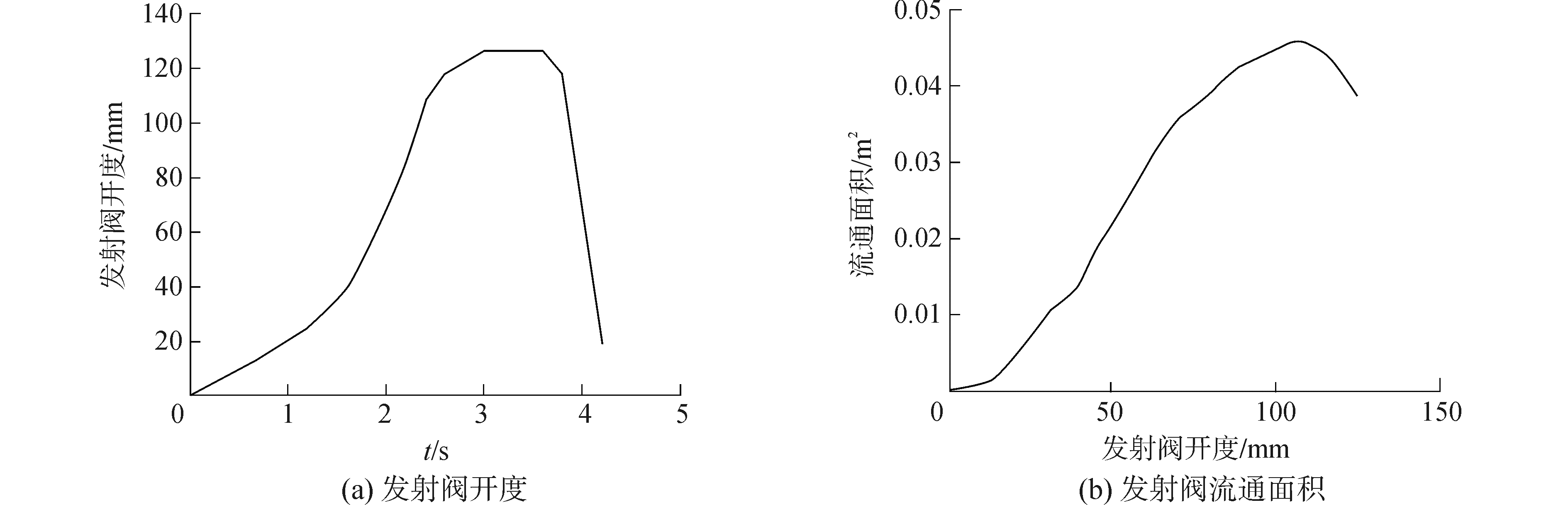Download: 图 3 发射阀模型 Fig. 3 The model of launch valves
2.1.3 气缸模型

1) 假设气缸与外界不存在热交换；

2) 气缸为刚性容器，忽略压强和温度导致的体积变化。

3) 气缸充汽过程为准静态过程，可以近似认为在每一时刻，气缸内热力学变化量都无限接近于一平衡态，满足状态方程。

4) 忽略气缸充汽过程中，高温高压蒸汽与气缸壁之间的摩擦损失。

5) 认为在储汽筒和气缸之间的管道中，高温高压蒸汽做绝热稳定流动。

 ${\rm{d}}Q = {{i'}_1}{\rm{d}}{M_s} = {\rm{d}}U + {\rm{d}}W$ (13)

 ${\rm{d}}U = {c_v}{\rm{d}}\left( {{T_1}{M_1}} \right)$ (14)

 ${\rm{d}}W = {P_1}{\rm{d}}{V_1}$ (15)

 ${{i'}_1}{\rm{d}}{M_s} = {c_p}{{T'}_1}{\rm{d}}{M_s}$ (16)

 ${c_p}{{T'}_1}{\rm{d}}{M_s} = {c_v}{\rm{d}}\left( {{T_1}{M_1}} \right) + {P_1}{\rm{d}}{V_1}$ (17)

 ${P_1}{V_1} = {M_1}{R_g}{T_1}$ (18)

 $\kappa {R_g}{{T'}_1}{\rm{d}}{M_s} = {V_1}{\rm{d}}{P_1} + \kappa {P_1}{\rm{d}}{V_1}$ (19)

 $\frac{{{\rm{d}}{P_1}}}{{{\rm{d}}t}} = \frac{{\kappa {R_g}{{T'}_1}{Q_{m1}}}}{{{V_1}}} - \frac{{\kappa {P_1}}}{{{V_1}}}\frac{{{\rm{d}}{V_1}}}{{{\rm{d}}t}}$ (20)
 ${V_1} = {V_{10}} + {A_1}X = {A_1}\left( {{X_{10}} + X} \right)$ (21)

 $\frac{{{\rm{d}}{P_1}}}{{{\rm{d}}t}} = \frac{{\kappa {R_g}{{T'}_1}{Q_{m1}}}}{{{A_1}\left( {{X_{10}} + X} \right)}} - \frac{{\kappa {P_1}}}{{{X_{10}} + X}}\frac{{{\rm{d}}X}}{{{\rm{d}}t}}$ (22)

 ${F_T} = 2\left( {{P_1}{A_1} - {P_2}{A_2}} \right)$ (23)

2.2 牵引释放装置数学模型 2.2.1 弹射杆数学模型

 ${T_1} = T\cos {\theta _c}$ (24)

 ${T_2} = {T_1}\tan {\theta _c}$ (25)
2.2.2 牵制杆数学模型

 ${R_{\min }} = 1.35 \times \left( {\frac{{P + f + 0.5G}}{{\cos \beta }}} \right)$ (26)

 ${R_1} = R\cos \beta$ (27)

 ${R_2} = {R_1}\tan \beta$ (28)
2.3 蒸汽弹射系统的动力学模型 2.3.1 建模假设

1) 不考虑甲板运动，即不考虑舰面纵摇、舰面横摇和舰面生沉运动；

2) 质量车为刚性车身，即不考虑质量车的变形；

3) 质量车质量集中在质心上，即质量车的质量被认为集中于一个假想点；

4) 不考虑质量车轮胎的压缩变形，轮胎受到弹射力和牵制力的作用会发生形变，本文忽略此形变；

5) 质量车弹射过程前处于完全对中状态，无偏航角，即弹射跑道与质量车的对称平面平行；

6) 弹射过程中，不考虑侧向载荷及湍流的影响，即不考虑侧向甲板风及湍流的影响；

7) 弹射过程中，弹射角和牵制角为定值，轮胎受到弹射力和牵制力的作用会发生形变，这样弹射角和牵制角也会发生相应的变化，本文忽略轮胎的形变，则弹射角和牵制角为定值；

8) 活塞为刚性体，即不考虑活塞的变形；

9) 活塞质量集中在质心上，即活塞的质量被认为集中于一个假想点。

2.3.2 施加预紧力阶段数学模型

1) 活塞施加预紧力阶段数学模型。

 ${F_Y} - f - {T_1} = 0$ (29)

 $f = \left\{ {\begin{array}{*{20}{c}} \begin{array}{l} \left( {{m_1}g - {T_1}\tan {\theta _c}} \right){\mu _2},\\ \left( {{T_1}\tan {\theta _c} - {m_1}g} \right){\mu _2}, \end{array}&\begin{array}{l} {m_1}g \ge {T_2}\\ {m_1}g < {T_2} \end{array} \end{array}} \right.$ (30)

 ${T_1} = \left\{ \begin{array}{l} \frac{{{F_Y} - {m_1}g{\mu _2}}}{{1 - {\mu _2}\tan {\theta _c}}},\;\;\;\;{m_1}g \ge {T_2}\\ \frac{{{F_Y} + {m_1}g{\mu _2}}}{{1 + {\mu _2}\tan {\theta _c}}}\;\;\;\;\;{m_1}g < {T_2} \end{array} \right.$ (31)

2) 质量车施加预紧力阶段数学模型。

 ${T_1} - {f_1} - {f_2} - {R_1} = 0$ (32)

 ${f_1} = {N_1}{\mu _1}$ (33)

 ${f_2} = {N_2}{\mu _1}$ (34)

 ${R_1} = \frac{{{T_1}\left( {1 - {\mu _1}\tan {\theta _c}} \right) - mg{\mu _1}}}{{{\mu _1}\tan \beta + 1}}$ (35)
2.3.3 弹射力加载阶段静力学模型

1) 活塞弹射力加载阶段静力学模型。

 ${F_Y} + {F_T} - f - {T_1} = 0$ (36)

2) 质量车弹射力加载阶段静力学模型。

 ${T_1} - {f_1} - {f_2} - {R_1} = 0$ (37)
2.3.4 弹射滑跑阶段动力学模型

1) 活塞弹射滑跑阶段动力学模型。

 ${F_T} - f - {T_1} = {m_1}{a_1}$ (38)

2) 质量车弹射滑跑阶段动力学模型。

 ${T_1} - {f_1} - {f_2} - D = ma$ (39)

 $D = \frac{1}{2}{C_D}A\rho {V^2}$ (40)

 $\begin{array}{l} a = \frac{{\left( {{F_T} + {m_1}g{\mu _2}} \right)\left( {1 - {\mu _1}\tan {\theta _c}} \right)}}{{m\left( {1 + {\mu _2}\tan {\theta _c}} \right) + {m_1}\left( {1 - {\mu _1}\tan {\theta _c}} \right)}} - \\ \;\;\;\;\frac{{\left( {D + G{\mu _1}} \right)\left( {1 + {\mu _2}\tan {\theta _c}} \right)}}{{m\left( {1 + {\mu _2}\tan {\theta _c}} \right) + {m_1}\left( {1 - {\mu _1}\tan {\theta _c}} \right)}} \end{array}$ (41)
3 质量车弹射系统算例分析

3.1 储汽筒压力 3.1.1 理论分析

3.1.2 仿真验证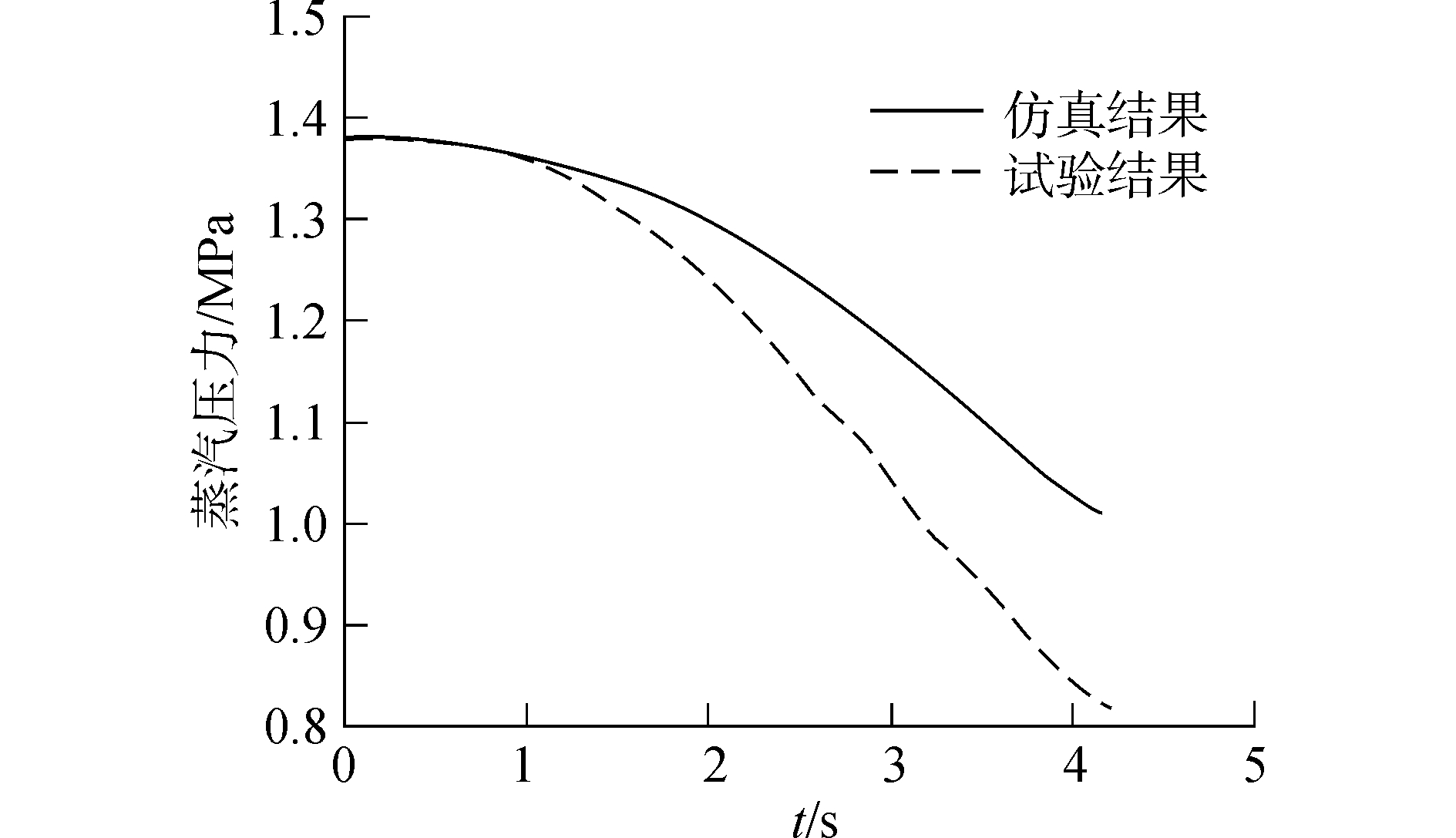Download: 图 4 储汽筒压力对比 Fig. 4 The contrast figure of accumulator pressure

1) 储汽筒内压力在整个弹射过程中呈不断减小的趋势。这同理论分析中的结果相符。

2) 由于仿真考虑的是理想情况，即储汽筒不与外界发生热交换，储汽筒放汽过程中，高温高压蒸汽与储汽筒壁之间没有摩擦损失，蒸汽管道及气缸没有蒸汽泄露等。仿真中储汽筒的压降低于试验中储汽筒的压降。

3.2 气缸压力 3.2.1 理论分析

3.2.2 仿真验证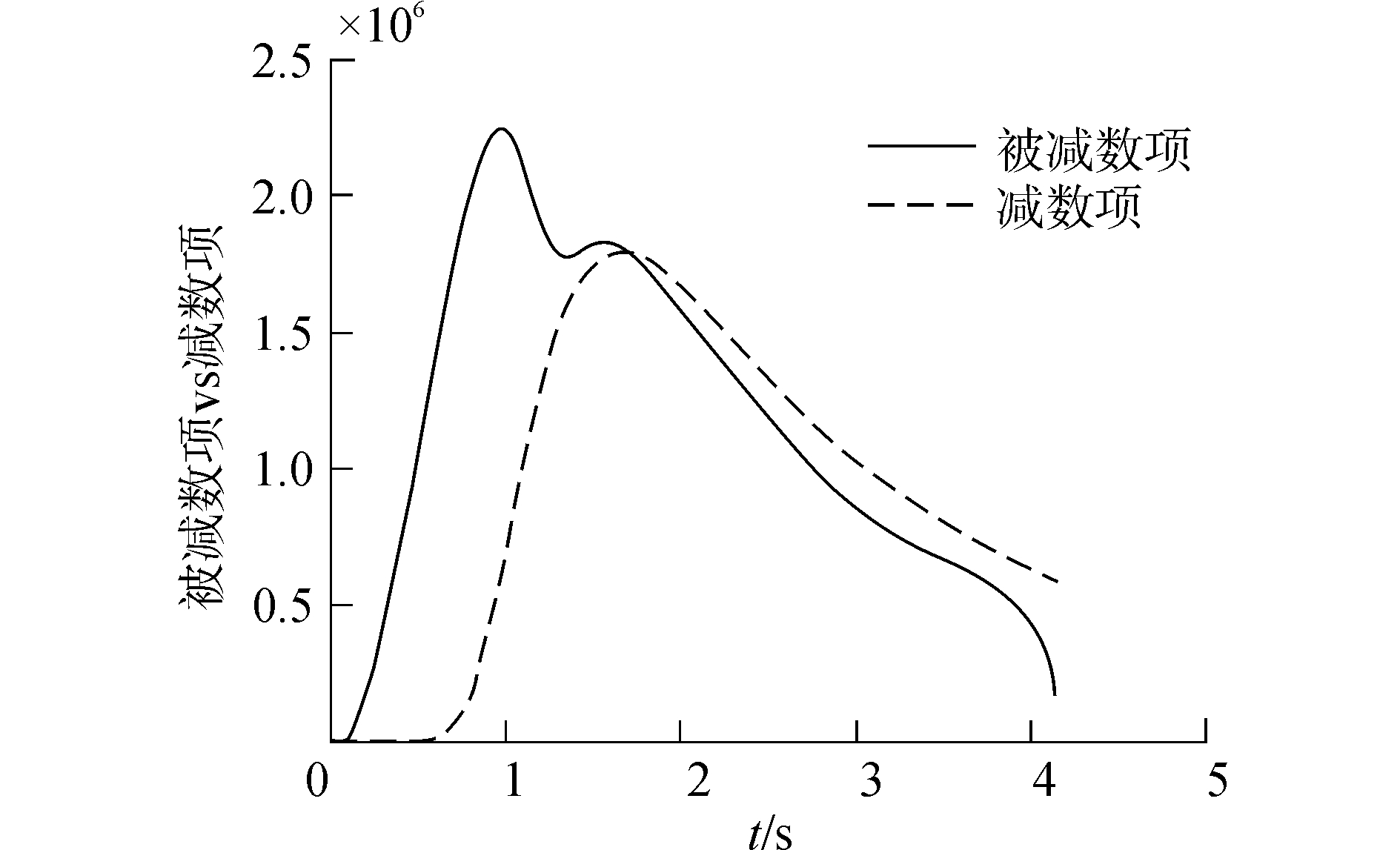Download: 图 5 被减数项与减数项对比 Fig. 5 The contrast figure of minuend and reduction item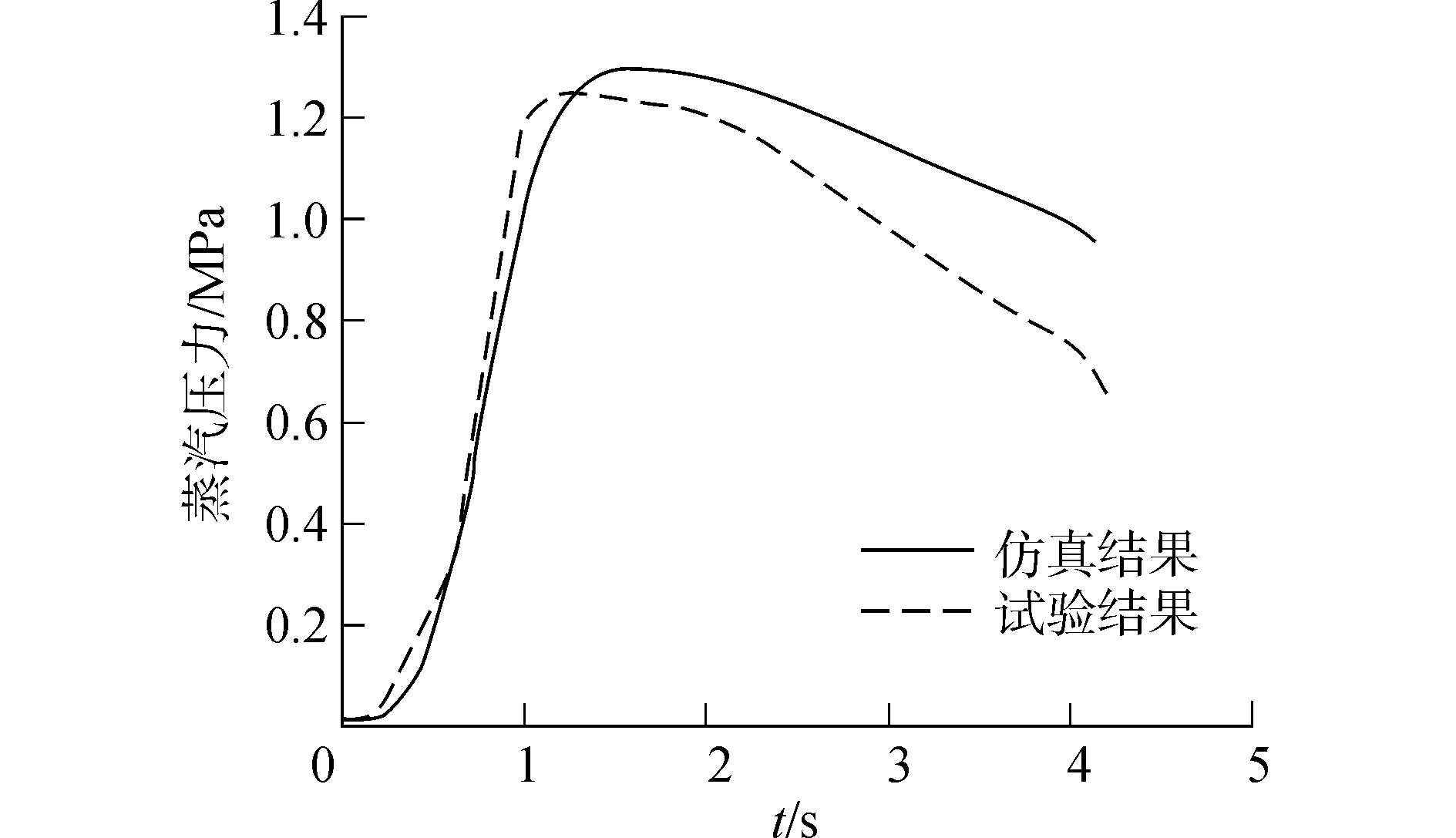Download: 图 6 气缸压力对比 Fig. 6 The contrast figure of cylinder pressure

1) 由减数项的仿真结果曲线可知，牵制杆突卸时刻为0.616 s。此后，活塞和质量车开始运动。

2) 在第1.702 s之前，减数项小于被减数项，气缸压力的导数大于0，气缸内压力持续增加；在第1.702 s以后，减数项开始大于被减数项，气缸内压力持续减小；

3) 气缸内压力与弹射力对应，可以通过设计被减数项和减数项来得到气缸压力，进而得到理想的弹射力。

3.3 质量车加速度 3.3.1 理论分析

3.3.2 仿真验证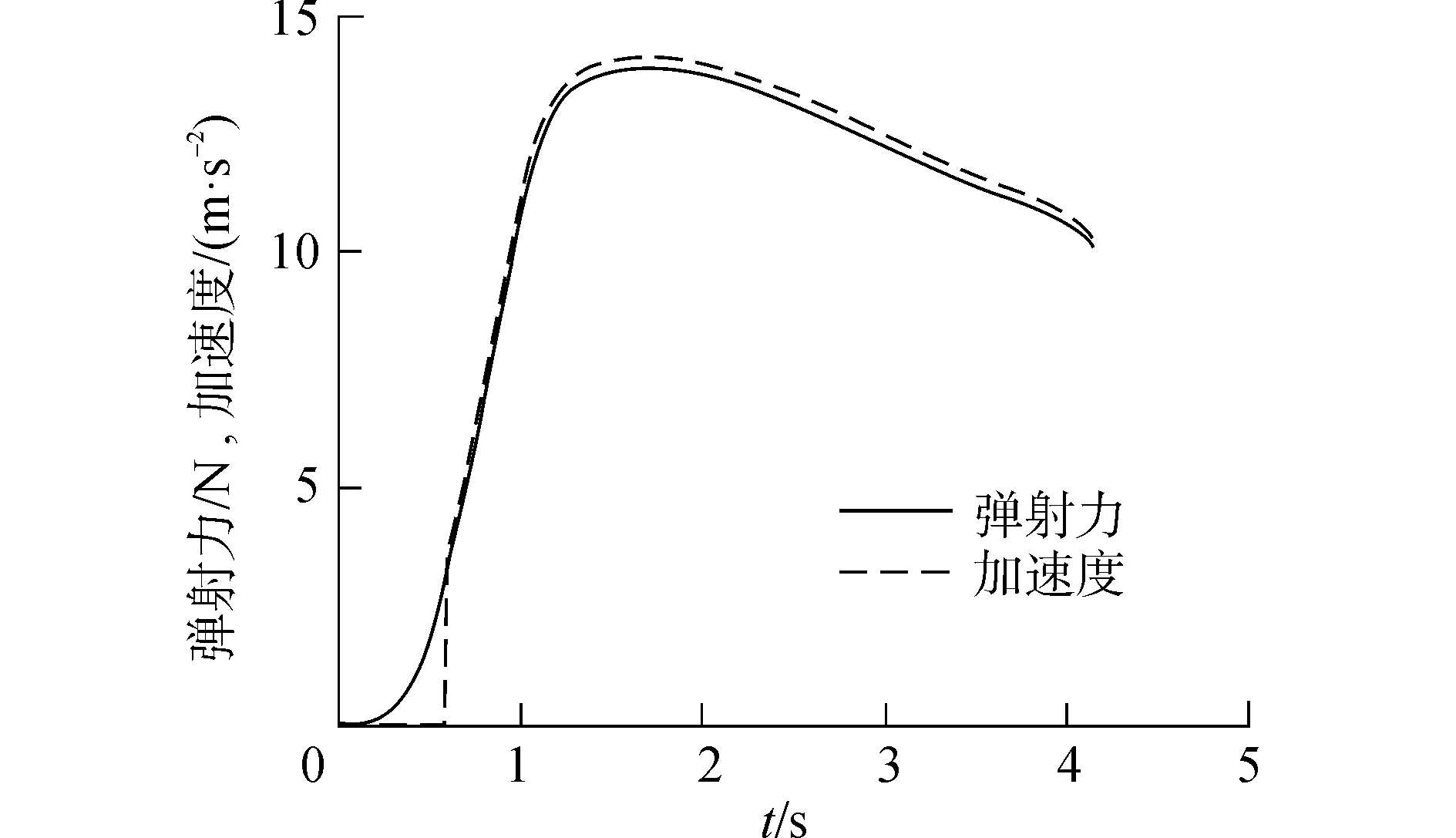Download: 图 7 弹射力与质量车加速度趋势对比 Fig. 7 The contrast figure of catapult force and deadload acceleration trend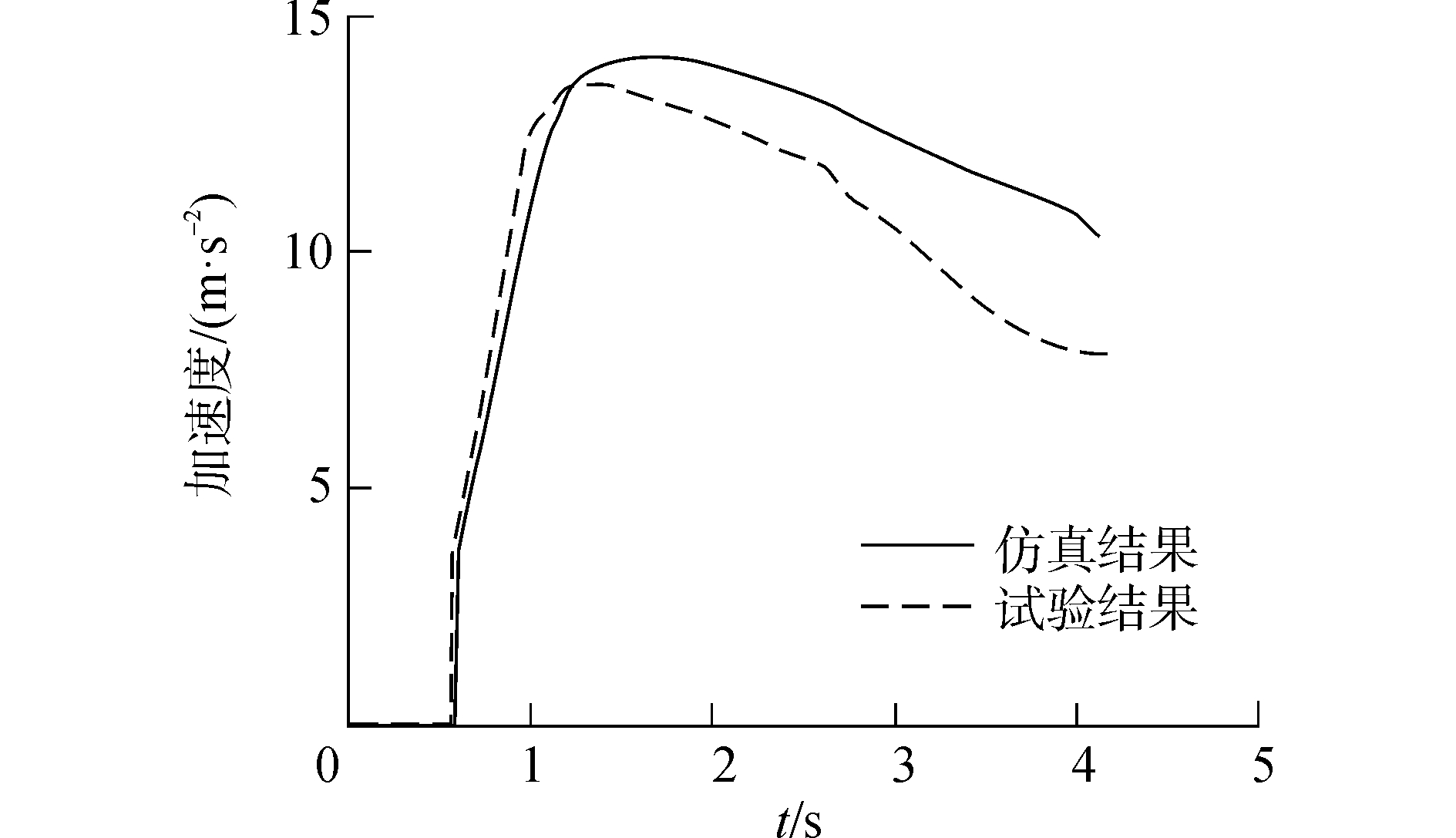Download: 图 8 质量车加速度对比 Fig. 8 The contrast figure of deadload acceleration

1) 弹射力曲线和质量车加速度的曲线趋势基本吻合，所以弹射力是质量车加速度变化的主要原因。

2) 由于弹射力由气缸压力主导，而在第1.702 s之前，气缸压力持续增加，导致质量车加速度持续增加；在第1.702 s以后，气缸内压力持续减小，导致质量车加速度持续减小。

3.4 蒸汽弹射系统受到的阻力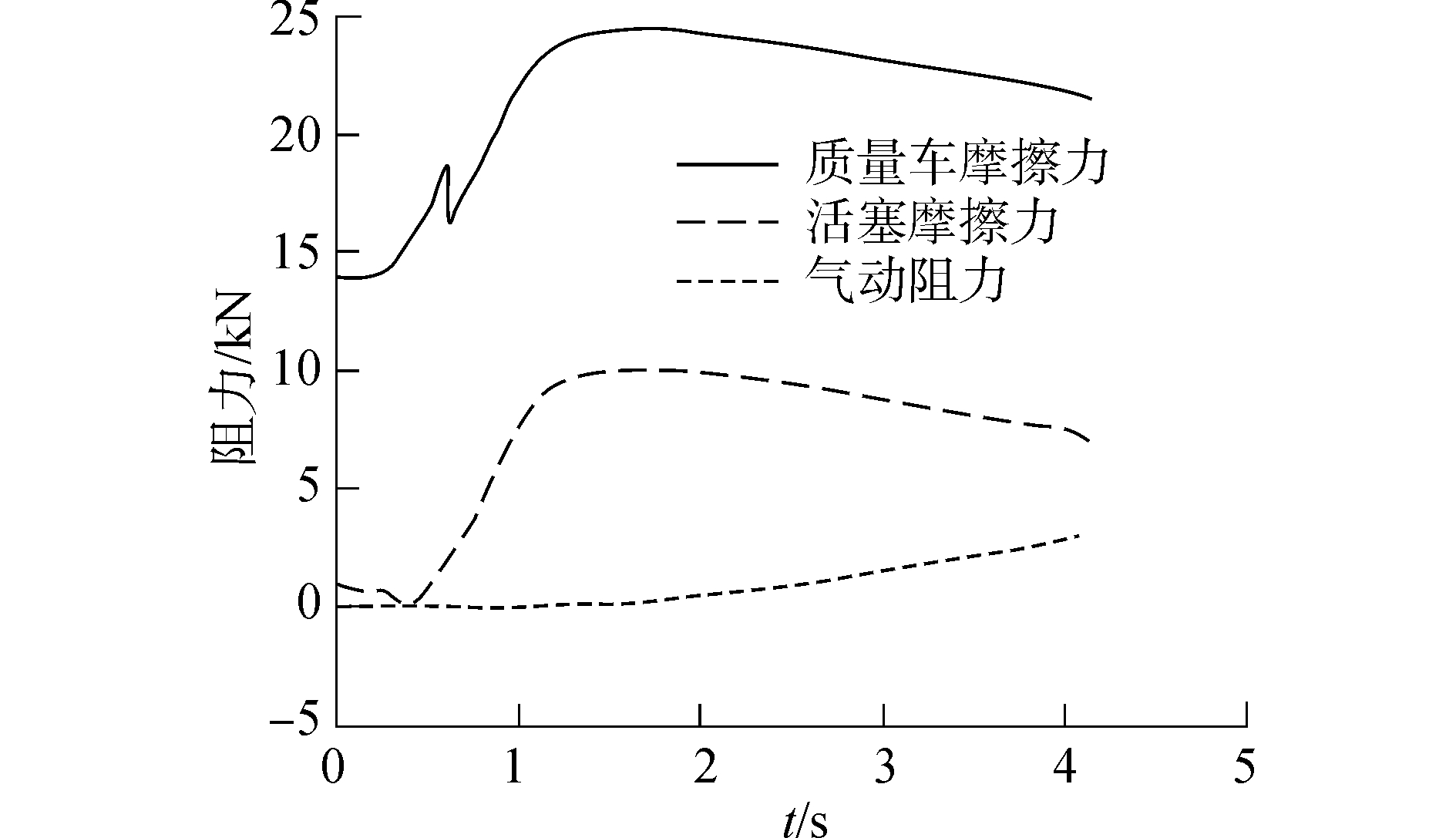Download: 图 9 蒸汽弹射系统受到的阻力 Fig. 9 The resistance of steam catapult system

1) 在蒸汽弹射系统受到的三个阻力当中，质量车受到的摩擦阻力最大，空气阻力最小。

2) 在牵制杆突卸时刻，由于牵制力和牵制杆轴向力的竖直分量突然消失，质量车摩擦阻力会突然减小，后来由于弹射杆轴向力逐渐增大，导致质量车摩擦力逐渐增大。

3) 由于弹射杆轴向力的竖直分量逐渐增加，导致活塞的摩擦力逐渐减小，直到在弹射力加载的某一时刻，弹射杆轴向力的竖直分量等于活塞的重力，此时活塞摩擦力为零，后来由于弹射杆轴向力逐渐增大，导致活塞摩擦力也逐渐增大。

3.5 蒸汽弹射器关键参数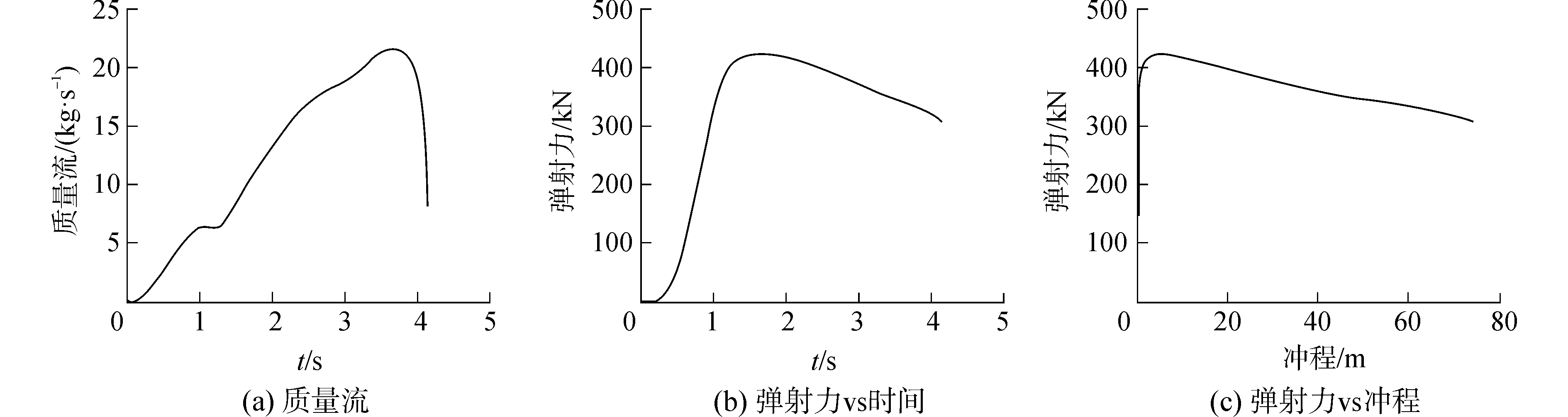Download: 图 10 蒸汽弹射器关键数据曲线 Fig. 10 The key data curve of steam catapult system

1) 质量流的变化趋势与发射阀开度的变化趋势一致。

2) 弹射力随冲程的变化曲线可以看作一个分段函数，即前一段斜率为负，后一段斜率为正的分段函数。

3.6 其他关键参数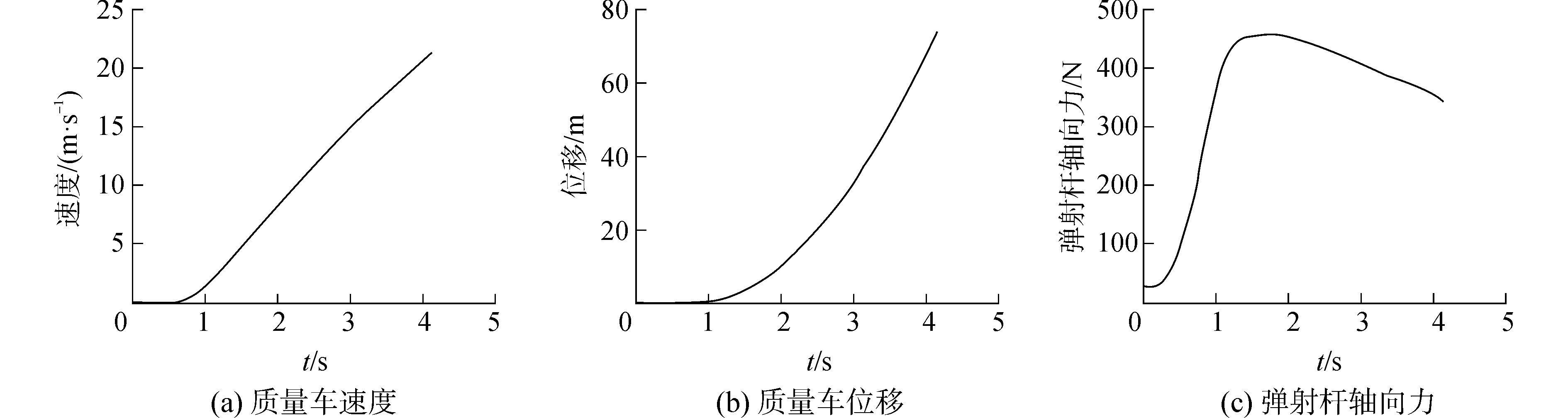Download: 图 11 其他关键数据曲线 Fig. 11 Other key data curve

1) 牵制力突卸之后，质量车的速度和位移逐渐增加。

2) 由于在发射阀开启之前，张紧系统会对蒸汽弹射系统施加预紧力，这导致弹射启动时刻，弹射杆就已经存在力；弹射杆轴向力与弹射力和气缸压力相关，且弹射杆轴向力的峰值大于弹射力的峰值。

4 结论

1) 通过对储汽筒压力、气缸压力和质量车加速度三个蒸汽弹射系统关键参数进行理论分析与仿真验证，并与实验数据进行对比，验证了本文建模方法的正确性。本文的仿真结果对蒸汽弹射系统的设计及实验提供了参考。

2) 通过建立包括施加预紧力阶段、弹射力加载阶段和弹射滑跑阶段的全过程质量车蒸汽弹射的仿真模型。这样更加符合工程实际情况。本文所建立的模型可对蒸汽弹射器、牵引释放装置和负载之间的相互作用关系研究提供参考。

3) 仿真结果表明：在蒸汽弹射系统的三个阻力当中，质量车摩擦力影响最大，空气阻力影响最小；弹射力与冲程的曲线可以看成前一段斜率为正，后一段斜率为负的分段函数；弹射杆轴向力的峰值大于弹射力的峰值。

  刘星宇, 许东松, 王立新. 滑跃起飞中机舰参数的适配性分析[J]. 北京航空航天大学学报, 2011, 37(6): 644-648, 653. LIU Xingyu, XU Dongsong, WANG Lixin. Match features of aircraft-carrier parameters during ski-jump takeoff[J]. Journal of Beijing University of Aeronautics and Astronautics, 2011, 37(6): 644-648, 653. (0)  DOYLE M R, SAMUEL D J, CONWAY T, et al. Electromagnetic aircraft launch system-EMALS[J]. IEEE transactions on magnetics, 1995, 31(1): 528-533. DOI:10.1109/20.364638 (0)  SLAVIN F J. Aircraft steam catapults[J]. Mechanical engineering, 1969, 91: 42-46. (0)  U.S. Naval Air Engineering Center. Steam catapult performance prediction program documentation[R]. AD704528, 1970. (0)  U.S. Naval Air Engineering Center. Evaluation of advanced method for steam catapult performance prediction with shipboard test data[R]. AD742804, 1972. (0)  NAVY. MIL-A-8863C, Airplane strength and rigidity ground loads for navy acquired airplanes[S]. New York: NAVY, 1993. (0)  MIL-STD-2066, Catapulting and arresting gear forcing functions for aircraft structural design[S]. New Jersey: Department of the Navy, 1982. (0)  SMALL D B. Full scale tests of nose tow catapulting[C]//Proceedings of the 1st AIAA Annual Meeting. Reston, 1964: 1-3. (0)  LUCAS C B. Catapult criteria for a carrier-based aircraft AD702814[R]. Washington DC: Defense Technical Information Center, 1968. (0)  赵险峰. 航母舰载机蒸汽弹射系统的数学仿真研究[J]. 舰载武器, 1996(3): 45-53. ZHAO Xianfeng. Study on the mathematical simulation of the steam catapult system of carrier-based aircraft[J]. Shipborne weapons, 1996(3): 45-53. (0)  余晓军, 高翔, 钟民军. 蒸汽弹射器的动力学仿真研究[J]. 船海工程, 2005(3): 1-4. YU Xiaojun, GAO Xiang, ZHONG Minjun. Simulation on dynamics of the steam-powered catapult[J]. Ship & ocean engineering, 2005(3): 1-4. DOI:10.3963/j.issn.1671-7953.2005.03.002 (0)  程刚, 倪何, 孙丰瑞. 舰载蒸汽弹射系统建模与仿真研究[J]. 武汉理工大学学报(交通科学与工程版), 2010, 34(2): 301-305. CHENG Gang, NI He, SUN Fengrui. Modeling and simulation research on naval steam-power aircraft launch system[J]. Journal of Wuhan University of Technology (transportation science & engineering), 2010, 34(2): 301-305. DOI:10.3963/j.issn.1006-2823.2010.02.022 (0)  张雷, 冯蕴雯, 薛小锋, 等. 舰载机蒸汽弹射动力学仿真分析[J]. 航空计算技术, 2012, 42(6): 29-33. ZHANG Lei, FENG Yunwen, XUE Xiaofeng, et al. Dynamics simulation of carrier-based aircraft steam catapult[J]. Aeronautical computing technique, 2012, 42(6): 29-33. DOI:10.3969/j.issn.1671-654X.2012.06.008 (0)  高俊东, 崔帅, 冯蕴雯, 等. 舰载机蒸汽弹射器热力学仿真分析[J]. 机械科学与技术, 2013, 32(7): 963-967. GAO Jundong, CUI Shuai, FENG Yunwen, et al. Simulation and analysis of thermodynamics for carrier-based aircraft steam catapult[J]. Mechanical science and technology for aerospace engineering, 2013, 32(7): 963-967. (0)  朱齐丹, 刘恒, 李晓琳. 舰载机偏心情况下弹射起飞研究[J]. 飞行力学, 2016, 34(2): 10-14. ZHU Qidan, LIU Heng, LI Xiaolin. Research on carrier-based aircraft catapult launching in the case of different eccentricity[J]. Flight dynamics, 2016, 34(2): 10-14. (0)  于浩, 聂宏. 舰载机偏中心定位弹射起飞弹射杆载荷分析[J]. 航空学报, 2010, 31(10): 1953-1959. YU Hao, NIE Hong. Launch bar load analysis of carrier-based aircraft during off-center catapult launch[J]. Acta aeronautica et astronautica sinica, 2010, 31(10): 1953-1959. (0)  刘星宇, 许东松, 王立新. 舰载飞机弹射起飞的机舰参数适配特性[J]. 航空学报, 2010, 31(1): 102-108. LIU Xingyu, XU Dongsong, WANG Lixin. Match characteristics of aircraft-carrier parameters during catapult takeoff of carrier-based aircraft[J]. Acta aeronautica et astronautica sinica, 2010, 31(1): 102-108. (0)